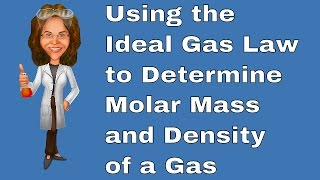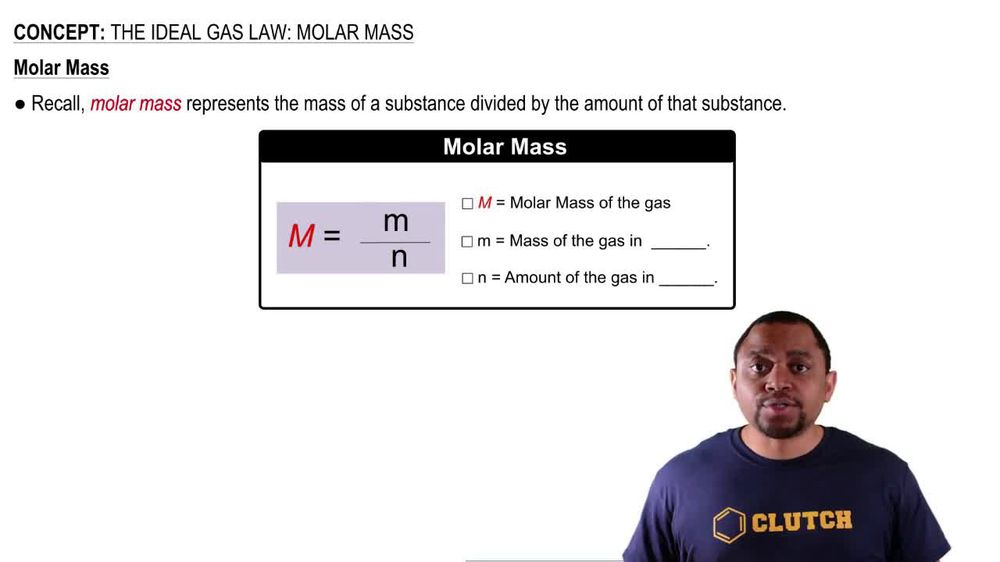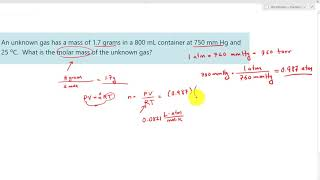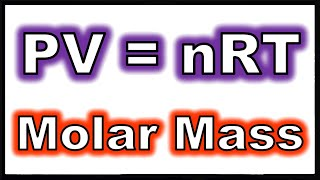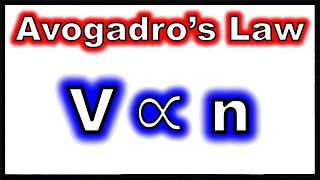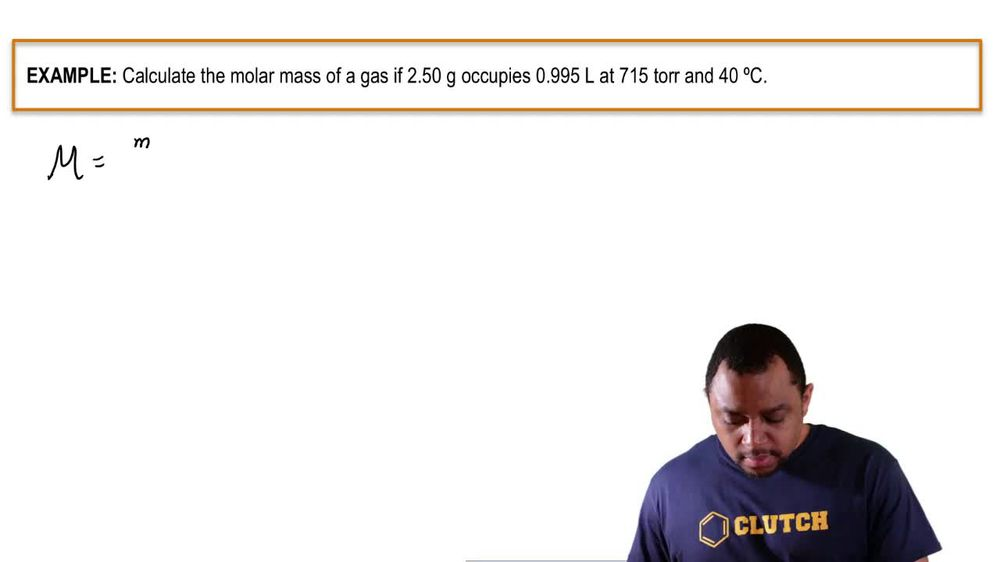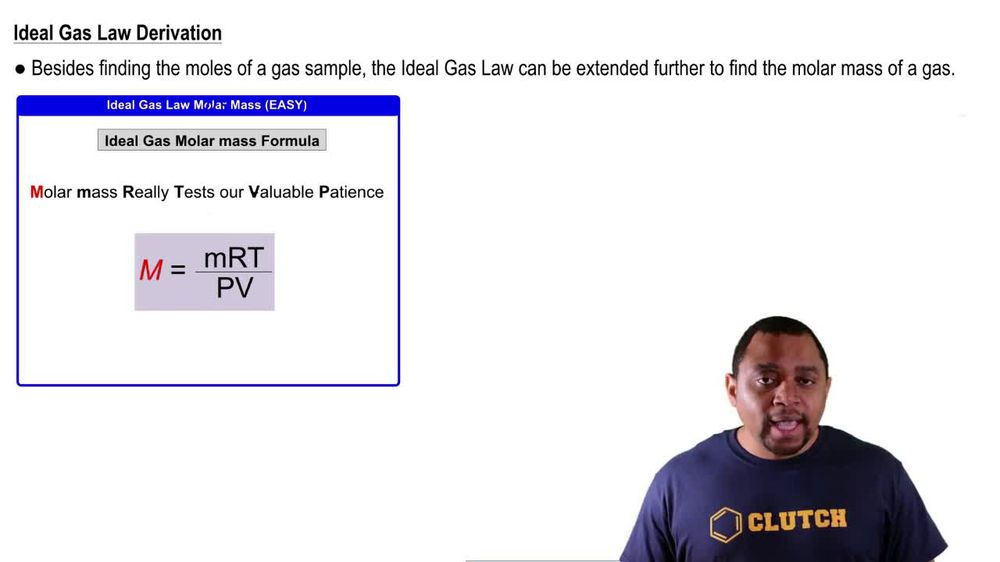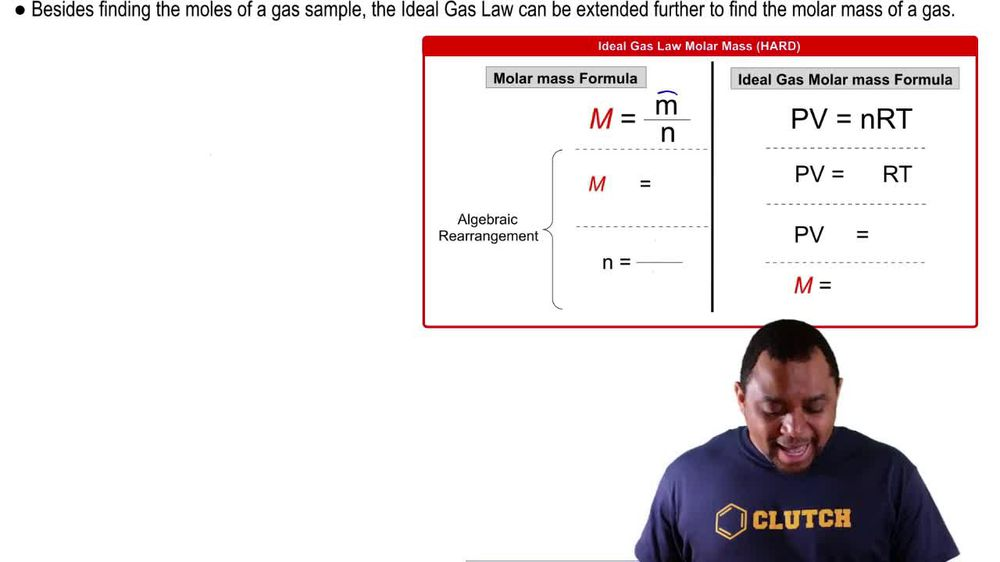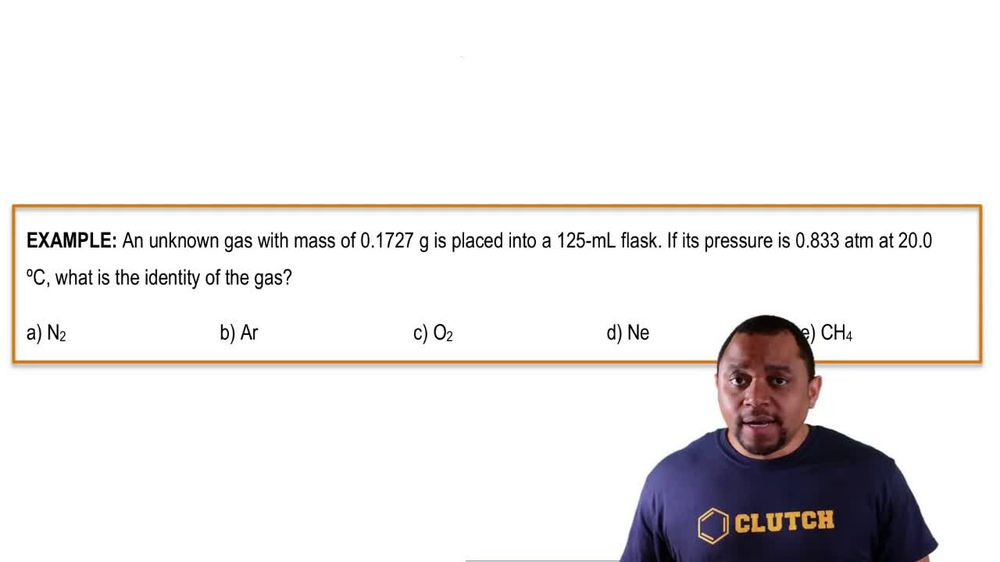Start typing, then use the up and down arrows to select an option from the list.
1. 7. Gases2. The Ideal Gas Law: Molar Mass# The Ideal Gas Law: Molar Mass Example 2

by Jules Bruno
87 views
0
here, it states in this example question An unknown gas with a mass of 0.1727 g is placed into millimeter flask. If it's pressure is 0.833 atmospheres at 20 degrees Celsius. What is the identity of the gas here? Were given different gasses. And we need to realize here that they're giving us our grams, which is mass. They're giving us volume. They're giving us pressure and they're giving us temperature with this Will be able to find the Moller Mass off are unknown. Gas. Then all we have to do is see which one has that Mueller Mass, um, as their actual weight. So we're gonna say here Mueller Mass equals rt over PV. We're gonna plug in the mass of our unknown. We're gonna plug in our, which is 0.8 to 06 leaders times atmospheres over moles. Times K. Remember, temperature has to be in Kelvin. So I had to 73.15 to this number to give us to 93.15 k divided by our pressure, which is 0.833 atmospheres and then our volume we convert those milliliters into leaders, so that gives us 0.1 to 5 leaders. Here we look and see what units cancel out and we see all that's left is grams over moles. So we have Moeller mass. When you plug that in, you get approximately 39 90 g promote. Yeah, All we have to do now is see which one of these gasses has a more mass closest to our answer. So here and to the two nitrogen is comes out to 28.2 g per mole are gone from the periodic table is approximately 39.95 g per mole. Oxygen is 32 g per mole grams per mole grams per mole grams per mole. Neon one neon is approximately 20 or so grams promote according to the periodic table, and then ch four is roughly around 16 g per mole. Our closest answer would have to be are gone. It's the one that's closest to this 39.90 g per mole. So here we've just utilized the molar mass version of the ideal gas law to find the molar mass of our unknown gas